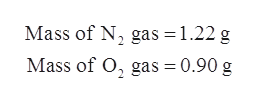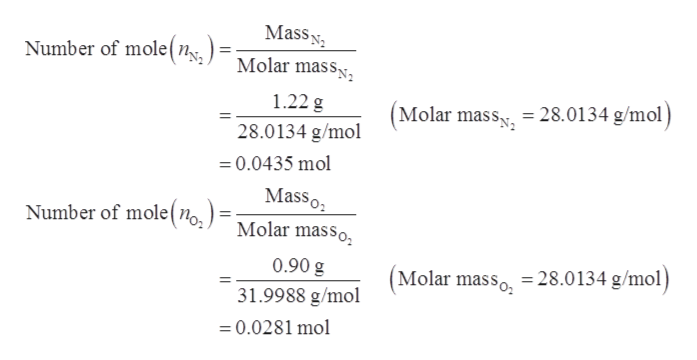# A gas mixture contains 1.22 g N2 and 0.90 g O2 in a 1.58-Lcontainer at 24 ∘C.Calculate the mole fraction of N2.

Question
16 views

A gas mixture contains 1.22 g N2 and 0.90 g O2 in a 1.58-Lcontainer at 24 ∘C.

Calculate the mole fraction of N2.

check_circle

Step 1

Mole fraction is one of the ways to express the concentration of solution. Mole fraction of a compound is defined as the ratio of number of moles of one compound to total number of moles.

Step 2

Given data:help_outlineImage TranscriptioncloseMass of N2 gas = 1.22 g Mass of O, gas = 0.90 g fullscreen
Step 3

Molar mass of N2 is 28.0134 g/mol.

Molar mass of O2 is 31.9988 g/mol.

The number of moles of N...help_outlineImage TranscriptioncloseMass Molar mass Number of mole(n 1.22 g (Molar mass, = 28.0134 g/mol 28.0134 g/mol 0.0435 mol Masso Molar masso Number of mole(n0 0.90 g 28.0134 g/mol) Molar masso 31.9988 g/mol =0.0281 mol fullscreen

### Want to see the full answer?

See Solution

#### Want to see this answer and more?

Solutions are written by subject experts who are available 24/7. Questions are typically answered within 1 hour.*

See Solution
*Response times may vary by subject and question.
Tagged in

### Physical Chemistry